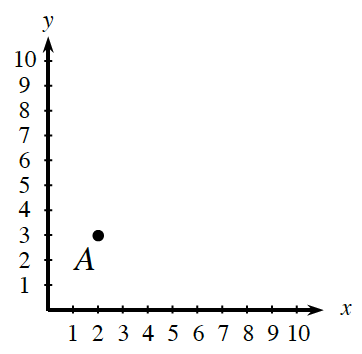### Home > ACC6 > Chapter 3 Unit 3 > Lesson CC1: 3.2.2 > Problem3-110

3-110.

Carefully copy the graph and point A on a piece of graph paper.

1. Plot the points listed below. Then connect them in the order given and connect point D to point A.
$A (2, 3), B (3, 7), C (7, 7), D (8, 3)$

2. What is the name of the shape that you drew?The shape is a quadrilateral with one pair of parallel sides of different lengths. Can you think of a name for this shape?

Trapezoid

Use the eTool below to plot the points.
Click the link at right for the full version of the eTool: